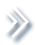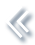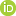####ArchivesReview Articles

## Prior-based Bayesian information criterion

M. J. Bayarri ,

Department of Statistics and Operations Research, University of Valencia, Valencia, SpainJames O. Berger ,

Department of Statistical Science, Duke University, Durham, NC, USAberger@stat.duke.edu,starf@ecnu.edu.cn

Woncheol Jang ,

Department of Statistics, Seoul National University, Seoul, KoreaSurajit Ray ,

School of Mathematics and Statistics, University of Glasgow, Glasgow, UKLuis R. Pericchi ,

Department of Mathematics, University of Puerto Rico, San Juan, Puerto RicoIngmar Visser

Department of Psychology, University of Amsterdam, Amsterdam, NetherlandsPages 2-13 | Received 24 Jun. 2017, Accepted 10 Feb. 2019, Published online: 14 Mar. 2019,
• Abstract
• References
• Citations

ABSTRACT

We present a new approach to model selection and Bayes factor determination, based on Laplace expansions (as in BIC), which we call Prior-based Bayes Information Criterion (PBIC). In this approach, the Laplace expansion is only done with the likelihood function, and then a suitable prior distribution is chosen to allow exact computation of the (approximate) marginal likelihood arising from the Laplace approximation and the prior. The result is a closed-form expression similar to BIC, but now involves a term arising from the prior distribution (which BIC ignores) and also incorporates the idea that different parameters can have different effective sample sizes (whereas BIC only allows one overall sample size n). We also consider a modification of PBIC which is more favourable to complex models.

## References

1. Bayarri, M. J., Berger, J. O., Forte, A., & García-Donato, G. (2012). Criteria for Bayesian model choice with application to variable selection. The Annals of Statistics40(3), 1550–1577. doi: 10.1214/12-AOS1013 [Crossref][Web of Science ®], [Google Scholar]
2. Berger, J. O. (1985). Statistical decision theory and Bayesian analysis (2nd ed.). New York: Springer-Verlag. [Crossref], [Google Scholar]
3. Berger, J. O., Bayarri, M. J., & Pericchi, L. R. (2014). The effective sample size. Econometric Reviews33, 197–217. doi: 10.1080/07474938.2013.807157 [Taylor & Francis Online][Web of Science ®], [Google Scholar]
4. Berger, J. O., Ghosh, J. K., & Mukhopadhyay, N. (2003). Approximations and consistency of bayes factors as model dimension grows. Journal of Statistical Planning and Inference112, 241–258. doi: 10.1016/S0378-3758(02)00336-1 [Crossref][Web of Science ®], [Google Scholar]
5. Berger, J. O., Pericchi, L. R., & Varshavsky, J. A. (1998). Bayes factors and marginal distributions in invariant situations. Sankhya: The Indian Journal of Statistics. Series A60, 307–321. [Google Scholar]
6. Berger, J. O., & Sellke, T. (1987). Testing a point null hypothesis: The irreconcilability of p values and evidence. Journal of the American statistical Association82, 112–122. [Taylor & Francis Online][Web of Science ®], [Google Scholar]
7. Bollen, K. A., Ray, S., Zavisca, J., & Harden, J. J. (2012). A comparison of Bayes factor approximation methods including two new methods. Sociological Methods and Research41, 294–324. doi: 10.1177/0049124112452393 [Crossref][Web of Science ®], [Google Scholar]
8. Chakrabarti, A., & Ghosh, J. K. (2006). A generalization of BIC for the general exponential family. Journal of Statistical Planning and Inference136(9), 2847–2872. doi: 10.1016/j.jspi.2005.01.005 [Crossref][Web of Science ®], [Google Scholar]
9. Drton, M., & Plummer, M. (2017). A Bayesian information criterion for singular models. Journal of the Royal Statistical Society: Series B (Statistical Methodology)79(2), 323–380. doi: 10.1111/rssb.12187 [Crossref][Web of Science ®], [Google Scholar]
10. Dudley, R. M., & Haughton, D. (1997). Information criteria for multiple data sets and restricted parameters. Statistica Sinica7, 265–284. [Web of Science ®], [Google Scholar]
11. Findley, D. F. (1991). Counterexamples to parsimony and BIC. Annals of the Institute of Statistical Mathematics43, 505–514. doi: 10.1007/BF00053369 [Crossref][Web of Science ®], [Google Scholar]
12. Foygel, R., & Drton, M. (2010). Extended Bayesian information criteria for Gaussian graphical models. In Advances in neural information processing systems (pp. 604–612). [Google Scholar]
13. Haughton, D. (1988). On the choice of a model to fit data from an exponential family. The Annals of Statistics16(1), 342–355. doi: 10.1214/aos/1176350709 [Crossref][Web of Science ®], [Google Scholar]
14. Haughton, D. (1991). Consistency of a class of information criteria for model selection in non-linear regression. Communications in Statistics: Theory and Methods20, 1619–1629. doi: 10.1080/03610929108830587 [Taylor & Francis Online][Web of Science ®], [Google Scholar]
15. Haughton, D. (1993). Consistency of a class of information criteria for model selection in nonlinear regression. Theory of Probability and its Applications37, 47–53. doi: 10.1137/1137009 [Crossref][Web of Science ®], [Google Scholar]
16. Haughton, D., Oud, J., & Jansen, R. (1997). Information and other criteria in structural equation model selection. Communications in Statistics, Part B – Simulation and Computation26(4), 1477–1516. doi: 10.1080/03610919708813451 [Taylor & Francis Online][Web of Science ®], [Google Scholar]
17. Jeffreys, H. (1961). Theory of probability. London: Oxford University Press. [Google Scholar]
18. Kass, R. E., & Raftery, A. (1995). Bayes factors. Journal of the American Statistical Association90, 773–795. doi: 10.1080/01621459.1995.10476572 [Taylor & Francis Online][Web of Science ®], [Google Scholar]
19. Kass, R. E., & Vaidyanathan, S. K. (1992). Approximate Bayes factors and orthogonal parameters, with application to testing equality of two binomial proportions. Journal of the Royal Statistical Society54, 129–144. [Google Scholar]
20. Kass, R. E., & Wasserman, L. (1995). A reference Bayesian test for nested hypotheses and its relationship to the Schwarz criterion. Journal of the American Statistical Association90, 928–934. doi: 10.1080/01621459.1995.10476592 [Taylor & Francis Online][Web of Science ®], [Google Scholar]
21. Pauler, D. (1998). The Schwarz criterion and related methods for normal linear models. Biometrika85, 13–27. doi: 10.1093/biomet/85.1.13 [Crossref][Web of Science ®], [Google Scholar]
22. Raftery, A. E. (1999). Bayes factors and BIC – comment on ‘A critique of the Bayesian information criterion for model selection’. Sociological Methods and Research27, 411–427. doi: 10.1177/0049124199027003005 [Crossref][Web of Science ®], [Google Scholar]
23. Schwarz, G. (1978). Estimating the dimension of a model. Annals of Statistics6, 461–464. doi: 10.1214/aos/1176344136 [Crossref][Web of Science ®], [Google Scholar]
24. Shen, G., & Ghosh, J. K. (2011). Developing a new BIC for detecting change-points. Journal of Statistical Planning and Inference141(4), 1436–1447. doi: 10.1016/j.jspi.2010.10.017 [Crossref][Web of Science ®], [Google Scholar]
25. Stone, M. (1979). Comments on model selection criteria of Akaike and Schwarz. Journal of the Royal Statistical Society, Series B41, 276–278. [Google Scholar]
26. Strawderman, W. E. (1971). Proper Bayes minimax estimators of the multivariate normal mean. The Annals of Mathematical Statistics42(1), 385–388. doi: 10.1214/aoms/1177693528 [Crossref], [Google Scholar]
27. Tierney, L., Kass, R. E., & Kadane, J. B. (1989). Fully exponential Laplace approximations to expectations and variances of nonpositive functions. Journal of the American Statistical Association84(407), 710–716. doi: 10.1080/01621459.1989.10478824 [Taylor & Francis Online][Web of Science ®], [Google Scholar]
28. Zak-Szatkowska, M., & Bogdan, M. (2011). Modified versions of Bayesian information criterion for sparse generalized linear models. Computational Statistics and Data Analysis55, 2908–2924. doi: 10.1016/j.csda.2011.04.016 [Crossref][Web of Science ®], [Google Scholar]